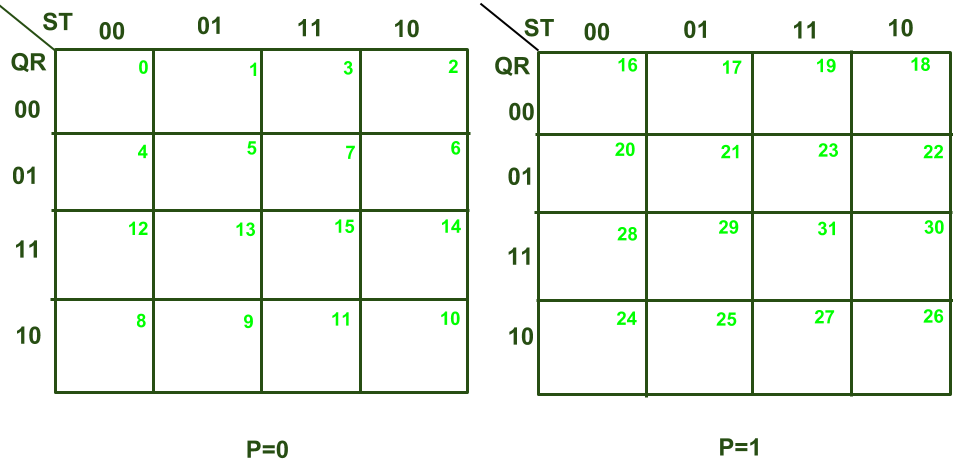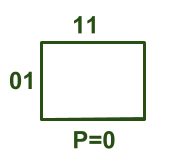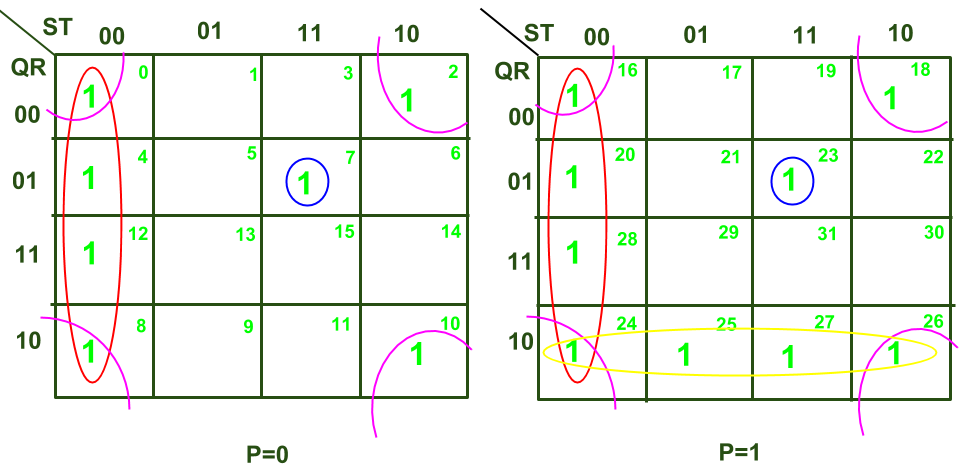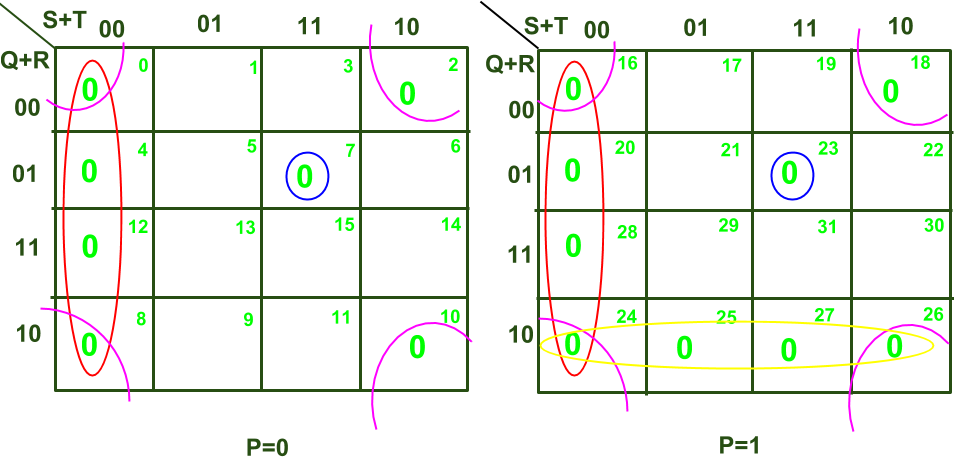# Digital Logic | 5 variable K-Map

Prerequisite – Implicant in K-Map
Karnaugh Map or K-Map is an alternative way to write truth table and is used for the simplification of Boolean Expressions. So far we are familiar with 3 variable K-Map & 4 variable K-Map. Now, let us discuss the 5-variable K-Map in detail.

Any Boolean Expression or Function comprising of 5 variables can be solved using the 5 variable K-Map. Such a 5 variable K-Map must contain= 32 cells . Let the 5-variable Boolean function be represented as :
f ( P Q R S T) where P, Q, R, S, T are the variables and P is the most significant bit variable and T is the least significant bit variable.

The structure of such a K-Map for SOP expression is given below :The cell no. written corresponding to each cell can be understood from the example described here:Here for variable P=0, we have Q = 0, R = 1, S = 1, T = 1 i.e. (PQRST)=(00111) . In decimal form, this is equivalent to 7. So, for the cell shown above the corresponding cell no. = 7. In a similar manner, we can write cell numbers corresponding to every cell as shown in the above figure.
Now let us discuss how to use a 5 variable K-Map to minimize a Boolean Function.

Rules to be followed :

1. If a function is given in compact canonical SOP(Sum of Products) form then we write “1” corresponding to each minterm ( provided in the question ) in the corresponding cell numbers. For eg:
Forwe will write “1” corresponding to cell numbers (0, 1, 5, 7, 30 and 31).

2. If a function is given in compact canonical POS(Product of Sums) form then we write “0” corresponding to each maxterm ( provided in the question ) in the corresponding cell numbers. For eg:
Forwe will write “0” corresponding to cell numbers (0, 1, 5, 7, 30 and 31).

Steps to be followed :

1. Make the largest possible size subcube covering all the marked 1’s in case of SOP or all marked 0’s in case of POS in the K-Map. It is important to note that each subcube can only contain terms in powers of 2 . Also a subcube ofcells is possible if and only if in that subcube for every cell we satisfy that “m” number of cells are adjacent cells .
2. All Essential Prime Implicants (EPIs) must be present in the minimal expressions.

I. Solving SOP function –
For clear understanding, let us solve the example of SOP function minimization of 5 Variable K-Map using the following expression :In the above K-Map we have 4 subcubes:

• Subcube 1: The one marked in red comprises of cells ( 0, 4, 8, 12, 16, 20, 24, 28)
• Subcube 2: The one marked in blue comprises of cells (7, 23)
• Subcube 3: The one marked in pink comprises of cells ( 0, 2, 8, 10, 16, 18, 24, 26)
• Subcube 4: The one marked in yellow comprises of cells (24, 25, 26, 27)

Now, while writing the minimal expression of each of the subcubes we will search for the literal that is common to all the cells present in that subcube.

• Subcube 1:• Subcube 2:• Subcube 3:• Subcube 4:Finally the minimal expression of the given boolean Function can be expressed as follows :II. Solving POS function –
Now, let us solve the example of POS function minimization of 5 Variable K-Map using the following expression :In the above K-Map we have 4 subcubes:

• Subcube 1: The one marked in red comprises of cells ( 0, 4, 8, 12, 16, 20, 24, 28)
• Subcube 2: The one marked in blue comprises of cells (7, 23)
• Subcube 3: The one marked in pink comprises of cells ( 0, 2, 8, 10, 16, 18, 24, 26)
• Subcube 4: The one marked in yellow comprises of cells (24, 25, 26, 27)

Now, while writing the minimal expression of each of the subcubes we will search for the literal that is common to all the cells present in that subcube.

• Subcube 1:• Subcube 2:• Subcube 3:• Subcube 4:Finally the minimal expression of the given boolean Function can be expressed as follows :NOTE:

1. For the 5 variable K-Map, the Range of the cell numbers will be from 0 to-1 i.e. 0 to 31.
2. The above mentioned term “Adjacent Cells” means that “any two cells which differ in only 1 variable”.My Personal Notes arrow_drop_upCheck out this Author's contributed articles.

If you like GeeksforGeeks and would like to contribute, you can also write an article using contribute.geeksforgeeks.org or mail your article to contribute@geeksforgeeks.org. See your article appearing on the GeeksforGeeks main page and help other Geeks.

Please Improve this article if you find anything incorrect by clicking on the "Improve Article" button below.

2

Please write to us at contribute@geeksforgeeks.org to report any issue with the above content.